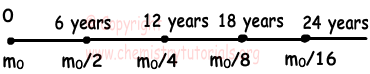EN | ES | DE | NL | RU

## Nuclear Chemistry (Radioactivity) Exam2 and Problem Solutions

Nuclear Chemistry (Radioactivity) Exam2 and Problem Solutions

1. If radioactive atom does one alpha and 2 beta decay, which ones of the following statements are true for this atom?

I. Its isotope is formed.

II. Its location in the periodic table does not change.

III. Its mass number decreases.

Solution:

If an atom does 1 alpha decay, its mass number decreases 4 and atomic number decreases 2. If an atom does 2 beta decay, mass number of it does not change but its atomic number increases 2.To sum up, mass number of this atom decreases 4 and atomic number does not change. Since number of protons does not change, location in the periodic table also does not change. Is mass number decreases and its isotope is formed. I, II and III are true.

2. Which ones of the following reactions are artificial radioactive decay?

I. 92238U → 90234Th + α

II. 1224Mg + β+1324Al

III. 49Be + 24He → 612C + n

Solution:

I. In this reaction 92238U does α decay and 90234Th is formed. It is natural decay.

II. In this reaction nonradioactive Mg atom does β+ and 1324Al is formed. It is artificial decay.

III. In this reaction nonradioactive Be atom does 24He decay and 612C is formed. It is also artificial decay.

3. Which ones of the following statements are true for given reaction?

49Be + 13H → 511B + 01n

I. It is fusion reaction

II. It is natural dasioactive decay

III. Total neutron number is conserved

Solution:

9Be and 13Hcome together and form 511B atom. Thus, it is artificial radioactive decay or fusion. I is true and II is false.

We write number of neutrons in both sides;

49Be + 13H → 511B + 01n

5 + 2 =6 + 1

7=7

We can say that number of neutrons in this reaction is conserved. III is true.

4. Which ones of the following statements are true for half life of radioactive matters?

I. It depends on amount of matter

II. It depends on types of matter

III. It depends on phase of matter

IV. It depends on temperature of matter

Solution:

Half life of radioactive matters depends on types of nucleus or neutron/proton ratio. Physical properties like amount of matter, temperature or phase do not affect half life. Isotopes of same atom can have different half life since there are changes in their nucleus and neutron/proton ratio. II is true ans I, III and IV are false.

5. If the decrease between 18. and 24. years in mass of radioactive matter is 4g, find its initial mass. Half life of this matter is 6 years.

Solution:

Let initial mass of matter be m0.We find difference between 18. and 24. years;

m0/8 - m0/16=4

m0/16=4

m0=64 g

The Original Author:

Tags: